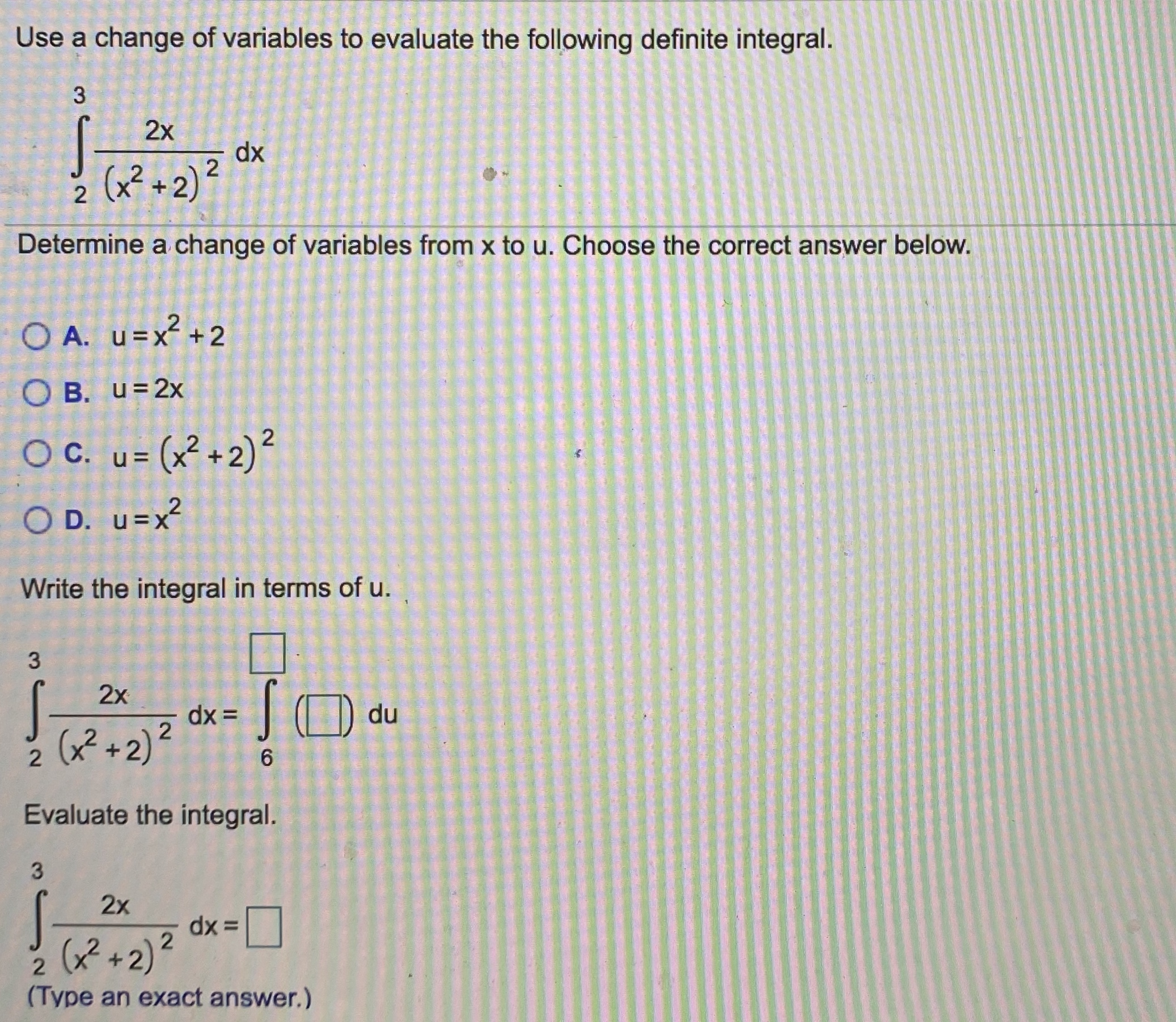# Determine a change of variables from x to u. Choose the correct answer below. O A. u=x+2 O B. u=2x OC. OC. u= (x²+2)² u = O D. u=x? Write the integral in terms of u. 2x |O du dx = (x² +2)? 6. Evaluate the integral. 3. 2x dx = (? +2)² (Type an exact answer.)

Question

9help_outlineImage TranscriptioncloseDetermine a change of variables from x to u. Choose the correct answer below. O A. u=x+2 O B. u=2x OC. OC. u= (x²+2)² u = O D. u=x? Write the integral in terms of u. 2x |O du dx = (x² +2)? 6. Evaluate the integral. 3. 2x dx = (? +2)² (Type an exact answer.) fullscreen

### Want to see this answer and more?

Experts are waiting 24/7 to provide step-by-step solutions in as fast as 30 minutes!*

*Response times vary by subject and question complexity. Median response time is 34 minutes and may be longer for new subjects.
Tagged in
MathCalculus

### Integration